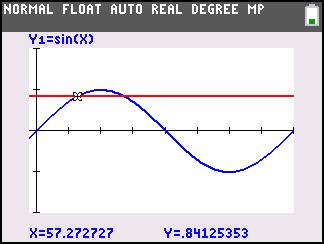### Solving Trigonometric Equations

Students represent and analyze mathematical situations and structures using algebraic symbols.
•TI-84 Plus CE
•TI-84 Plus C Silver Edition
• TI-84 Plus Silver Edition
• TI-84 Plus### Ain't No River Wide Enough

Students simulate the process a surveyor would use to measure the width of a river by measuring length on one side of the river and angles formed by various reference points.
•TI-84 Plus CE
•TI-84 Plus C Silver Edition
• TI-84 Plus Silver Edition
• TI-84 PlusStudents use a nomograph, or type of function machine, to determine the rule of several functions by entering values of x and observing how the value of y changes.
• TI-84 Plus Silver Edition
• TI-84 Plus### Proof of Identity

Students use graphs to verify the reciprocal identities. They then use the calculator's manual graph manipulation feature to discover the negative angle, cofunction, and Pythagorean trigonometric identities.
• TI-84 Plus Silver Edition
• TI-84 Plus### Getting Triggy With It

Students systematically explore the effect of the coefficients on the graph of sine or cosine functions.
•TI-84 Plus CE
•TI-84 Plus C Silver Edition
• TI-84 Plus Silver Edition
• TI-84 Plus••